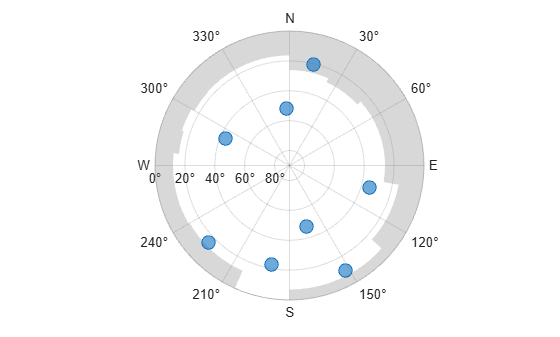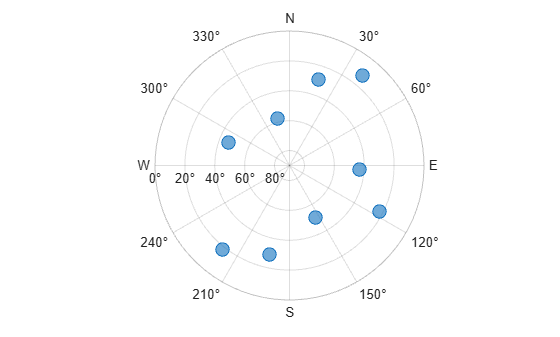# skyplot

Plot satellite azimuth and elevation data

## Syntax

``skyplot(azdata,eldata)``
``skyplot(azdata,eldata,labeldata)``
``skyplot(status)``
``skyplot(___,Name,Value)``
``skyplot(parent,___)``
``h = skyplot(___)``

## Description

example

````skyplot(azdata,eldata)` creates a sky plot using the azimuth and elevation data specified as vectors in degrees. Azimuth angles are measured in degrees, clockwise-positive from the North direction. Elevation angles are measured from the horizon line with 90 degrees being directly up. For details about the sky plot figure elements, see Main Sky Plot Elements.```
````skyplot(azdata,eldata,labeldata)` specifies data labels as a string array with elements corresponding to each data point in the `azdata` and `eldata` inputs.```
````skyplot(status)` specifies the azimuth and elevation data in a structure with fields `SatelliteAzimuth` and `SatelliteElevation`.```

example

````skyplot(___,Name,Value)` specifies options using one or more name-value arguments in addition to the input arguments in previous syntaxes. The name-value arguments are properties of the `SkyPlotChart` object. For a list of properties, see SkyPlotChart Properties.```
````skyplot(parent,___)` creates the sky plot in the figure, panel, or tab specified by `parent`.```
````h = skyplot(___)` returns the sky plot as a `SkyPlotChart` object, `h`. Use `h` to modify the properties of the chart after creating it. For a list of properties, see SkyPlotChart Properties.```

## Examples

collapse all

Create a GNSS sensor model as a `gnssSensor` System Object™.

`gnss = gnssSensor; `

Specify the position and velocity of the sensor. Simulate the sensor readings and get status from visible satellites. Store the azimuth and elevation angles as vectors.

```pos = [0 0 0]; vel = [0 0 0]; [~, ~, status] = gnss(pos, vel); satAz = status.SatelliteAzimuth; satEl = status.SatelliteElevation; ```

Plot the satellite postions.

`skyplot(satAz,satEl) `Animate the trajectory of satellite positions over time from a GNSS sensor.

Initialize the sky plot figure. Specify the relevant time-stepping information.

`skyplotHandle = skyplot(0,0); ````numHours = 12; dt = 100; numSeconds = numHours * 60 * 60; numSimSteps = numSeconds/dt;```

Create a GNSS sensor model as a `gnssSensor` System Object™.

`gnss = gnssSensor('SampleRate', 1/dt); `

Iterate through the time steps and do the following:

• Simulate the sensor readings. Specify the zero postion and velocity for the stationary sensor.

• Store the azimuth and elevation angles as vectors.

• Set the `AzimuthData` and `ElevationData` properties of the `SkyPlotChart` handle directly.

```for i = 1:numSimSteps [~, ~, status] = gnss([0 0 0],[0 0 0]); satAz = status.SatelliteAzimuth; satEl = status.SatelliteElevation; set(skyplotHandle,'AzimuthData',satAz,'ElevationData',satEl); drawnow end ```Load the azimuth and elevation data from a logfile generated by an Adafruit® GPS satellite sensor. The data provided in this example contains the azimuth and elevation of each satellite and the pseudorandom noise (PRN) codes. Store these values as vectors.

```load('gpsHWInfo','hwInfo') satAz = hwInfo.SatelliteAzimuths; satEl = hwInfo.SatelliteElevations; prn = hwInfo.SatellitePRNs; ```

Separate the satellites based on the PRN codes. To correlate each position with a group, create a `categorical` array. For this set of satellites, only the ones with PRNs less than 32 are used in the positioning solution.

```isUnused = (prn > 32); group = categorical(isUnused,[false true],["Used in Positioning Solution" "Unused"]);```

Visualize the satellites and specify the categorical groups in the `GroupData` name-value argument. Specify the PRN as the label for each point. Show the legend.

```skyplot(satAz,satEl,prn,GroupData=group) legend('Used','Unused')```## Input Arguments

collapse all

Azimuth angles for visible satellite positions, specified as an n-element vector of angles. n is the number of visible satellite positions in the plot. Azimuth angles are measured in degrees, clockwise-positive from the North direction.

Example: `[25 45 182 356]`

Data Types: `double`

Elevation angles for visible satellite positions, specified as an n-element vector of angles. n is the number of visible satellite positions in the plot. Elevation angles are measured from the horizon line with 90 degrees being directly up.

Example: `[45 90 27 74]`

Data Types: `double`

Labels for visible satellite positions, specified as an n-element string array. n is the number of visible satellite positions in the plot.

Example: `["G1" "G11" "G7" "G3"]`

Data Types: `string`

Satellite status, specified as a structure array with fields `SatelliteAzimuth` and `SatelliteElevation`. Typically, this status structure comes from a `gnssSensor` object, which simulates satellite positions and velocities.

Example: ```gnss = gnssSensor; [~,~,status] = gnss(position,velocity)```

Data Types: `struct`

Parent container, specified as a `Figure`, `Panel`, `Tab`, `TiledChartLayout`, or `GridLayout` object.

## Output Arguments

collapse all

Sky plot chart, returned as a `SkyplotChart` object, which is a standalone visualization. Use `h` to set properties on the sky plot chart. For more information, see SkyPlotChart Properties.

collapse all

### Main Sky Plot ElementsThe main elements of the figure are:

• Azimuth axes — Specified by the `azdata` input argument, azimuth angle positions are measured clockwise-positive from the North direction.

• Elevation axes —Specified by the `eldata` input argument, elevation angle positions are measured from the horizon line with 90 degrees being directly up.

• Labels — Specified by the `labeldata` input argument as a string array with an element for each point in the `azdata` and `eldata` vectors.

• Groups — Specified by the GroupData property, a `categorical` array defines the group for each satellite position.

### Standalone Visualization

A standalone visualization is a chart designed for a special purpose that works independently from other charts. Unlike other charts such as `plot` and `surf`, a standalone visualization has a preconfigured axes object built into it, and some customizations are not available. A standalone visualization also has these characteristics:

• It cannot be combined with other graphics elements, such as lines, patches, or surfaces. Thus, the `hold` command is not supported.

• The `gca` function can return the chart object as the current axes.

• You can pass the chart object to many MATLAB® functions that accept an axes object as an input argument. For example, you can pass the chart object to the `title` function.

## Version History

Introduced in R2021a